# Easy 3 Steps of Laplace Transform Circuit Element ModelsLaplace transform circuit element models is one of the Laplace transform applications. It converts the time-domain variable of the circuit elements into s-domain for Laplace transform analysis purpose.

Contents

Having mastered how to obtain the Laplace transform and its inverse, we are now prepared to employ the Laplace transform to analyze circuits.

## Laplace Transform Circuit Element Models

This usually involves three steps.

Steps in Applying the Laplace Transform:
1. Transform the circuit from the time domain to the s-domain.
2. Solve the circuit using nodal analysis, mesh analysis, source transformation, superposition, or any circuit analysis technique with which we are familiar.
3. Take the inverse transform of the solution and thus obtain the solution in the time domain.

Only the first step is new and will be discussed here. As we did in phasor analysis, we transform a circuit in the time domain to the frequency or s-domain by Laplace transforming each term in the circuit.

For a resistor, the voltage-current relationship in the time domain is

Taking the Laplace transform, we get

For an inductor,

Taking the Laplace transform of both sides gives

or

The s-domain equivalents are shown in Figure.(1), where the initial condition is modelled as a voltage or current source.

For a capacitor,

which transforms into the s-domain as

or

The s-domain equivalents are shown in Figure.(2). With the s-domain equivalents, the Laplace transform can be used readily to solve first- and second-order circuits.

We should observe from Equations.(3) to (8) that the initial conditions are part of the transformation. This is one advantage of using the Laplace transform in circuit analysis. Another advantage is that a complete response—transient and steady-state—of a network is obtained.

If we assume zero initial conditions for the inductor and the capacitor, the above equations reduce to:

The s-domain equivalents are shown in Figure.(3).

We define the impedance in the s-domain as the ratio of the voltage transform to the current transform under zero initial conditions; that is,

Thus, the impedances of the three circuit elements are

Table.(1) summarizes these. Assuming zero initial conditions

The admittance in the s-domain is the reciprocal of the impedance, or

The use of the Laplace transform in circuit analysis facilitates the use of various signal sources such as impulse, step, ramp, exponential, and sinusoidal.

The models for dependent sources and op amps are easy to develop drawing from the simple fact that if the Laplace transform of f(t) is F(s), then the Laplace transform of af(t) is aF(s)—the linearity property.

The dependent source model is a little easier in that we deal with a single value. The dependent source can have only two controlling values, a constant time either a voltage or a current. Thus,

The ideal op-amp can be treated just like a resistor. Nothing within an op-amp, either real or ideal, does anything more than multiplying a voltage by a constant.

Thus, we only need to write the equations as we always do use the constraint that the input voltage to the op-amp has to be zero and the input current has to be zero.

## Laplace Transform Circuit Element Models Examples

Let’s review the Laplace transform circuit element models examples below:

### Laplace Transform Circuit Element Models Example 1

Find in the circuit of Figure.(4), assuming zero initial condition

Solution:
We first transform the circuit from the time domain to the s-domain.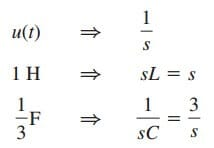The resulting s-domain circuit is in Figure.(5).

We now apply mesh analysis. For mesh 1,

For mesh 2,

Substituting this into Equation.(1.1),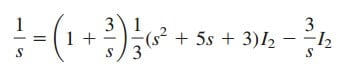Multiplying through by 3s gives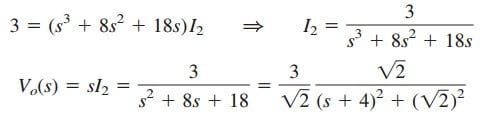Taking the inverse transform yields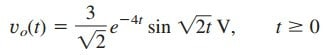### Laplace Transform Circuit Element Models Example 2

Find vo(t) in the circuit of Figure.(6). Assume vo(0) = 5 V.

Solution:
We transform the circuit to the s domain as shown in Figure.(7).

The initial condition is included in the form of the current source
C vo(0) = 0.1(5) = 0.5 A [See Figure.(2c)].

We apply nodal analysis. At the top node,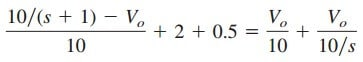orMultiplying through by 10,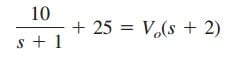or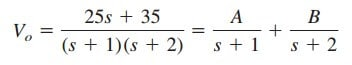where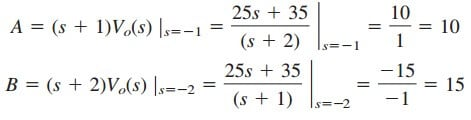Thus,Taking the inverse Laplace transform, we obtain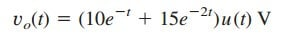### Laplace Transform Circuit Element Models Example 3

In the circuit in Figure.(8a), the switch moves from position a to position b at t = 0. Find i(t) for t > 0.

Solution:
The initial current through the inductor is i(0) = Io. For t > 0, Figure.(8a) shows the circuit transformed to the s domain. The initial condition is incorporated in the form of a voltage source as Li(0) = LIo.

Using mesh analysis,

or

Applying partial fraction expansion on the second term on the right-hand side of Equation.(4.2) yields

The inverse Laplace transform of this gives

where τ = R/L. The term in fences is the transient response, while the second term is the steady-state response. In other words, the final value is i() = Vo/R, which we could have predicted by applying the final-value theorem on Equations.(4.2) or (4.3); that is,